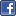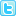# Abstract

## Reliability of period of gestation determined by ultrasound scan measurements

Objective: To determine reliability of period of gestation determined by three independent raters using four different foetal measurements.

Methods: One hundred and eighty pregnant women were divided into three equal groups. Each group was assigned a rater to perform ultrasound scan to measure bi-parietal diameter, femur length, abdominal circumference and head circumference and to compute the respective periods of gestation using these four measurements. Reliability between periods of gestation derived by each rater from above four measurements were analysed using repeated measure ANOVA. Results were expressed as intra-class correlation coefficients (ICCs) and coefficients of variation (CsOV).

Results: For Raters I (F= 6.47; p=0.001) and II, (F= 4.80; p= 0.003), computations using abdominal circumferences resulted in the lowest mean periods of gestation (PsOG). For Rater III, computations using both femur length and abdominal circumference resulted in the lowest mean periods of gestation (F= 7.5; p=0.001). ICCs were 0.73 (95%CI 0.64–0.81) for Rater I, 0.78 (95% CI 0.70–0.85) for Rater II and 0.87 (95% CI 0.81– 0.91) for Rater III When comparing CsOV, the highest variation for Raters I and III was observed for femur length. For Rater II it was bi-parietal diameter. The lowest variation for Rater I was observed for head circumference and for Raters II and III for abdominal circumference. The highest CsOV of all the PsOG were demonstrated by Rater III. When comparing the differences between the highest and the lowest values for each period of gestation determined, the difference was more than two weeks for 38% (n=23), 24% (n=14) and 22% (n=13) of observations made by Raters I, II and III respectively.

Conclusions: Reliability of period of gestation depends on the type of measurement taken, method of assessment and the rater who performs the measurements. Our findings are not conclusive enough to recommend any PsOG based on specific measurement more reliable than others. In-service training of the obstetricians is likely to improve the reliability of PsOG determined using ultra sound scan measurements.

Author(s): Chrishantha Abeysena , Pushpa Jayawardana

Abstract | Full-Text | PDF

Share this• ### 11th World Congress on Rheumatology, Orthopedics & Sports Medicine

Amsterdam, Netherlands

Miami, USA